### Importance sampling visible wavelength

Introduction

It has been half a year since my last post. Due to the pandemic and political environment in Hong Kong, I don't have much time/mood to work on my hobby path tracer... And until recently, I tried to get back to this hobby, may be it is better to work on some small tasks first. One thing I am not satisfied in previous spectral path tracing post is using 3 different cosh curves(with peak at the XYZ color matching functions) to importance sample the visible wavelength instead of 1. So I decided to revise it and find another PDF to take random wavelength samples. A demo with the updated importance sampled wavelength can be downloaded here and the python code used for generating the PDF can be viewed here (inspired by Bart Wronski's tweet to use Colab).

First Failed Try

The basic idea is to create a function with peak values at the same location as the color matching functions. I decided to use the sum of the XYZ color matching curves as PDF.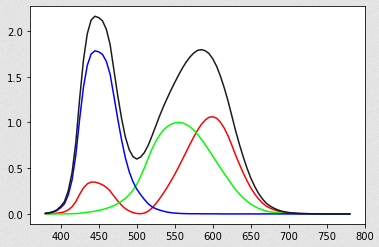Black line is the Sum of XYZ curves

To simplify calculation, analytical approximation of the XYZ curves can be used. A common approximation can be found here, which seems hard to be integrated(due to the λ square) to create the CDF. So a rational function is used instead:

The X curve is approximated with 1 peak and dropped the small peak at around 450nm, because we want to compute the "sum of XYZ" curve, that missing peak can be compensated by scaling the Z curves. The approximated curves looks like: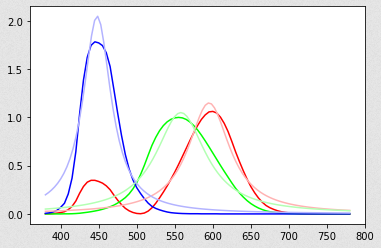Light colored curves are the approximated function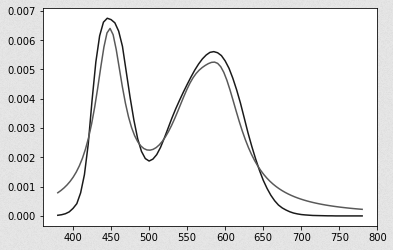Grey color curve is the approximated PDF
The approximated PDF is roughly similar to the sum of XYZ color matching curves. But I have made a mistake: Although the rational function can be integrated to create the CDF, I don't know how to compute the inverse of CDF(which is needed by the inverse method to draw random samples using uniform random numbers). So I need to find another way...

Second Try

From previous section, although I don't know how to find the inverse of the approximated CDF, out of curiosity, I still want to know how the approximated CDF looks like, so I plot the graph: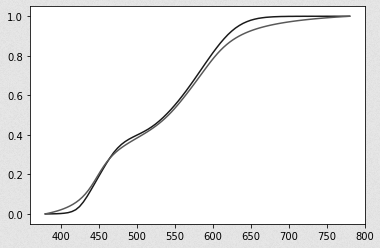Black line is the original CDFGrey line is the approximated CDF

It looks like some smoothstep functions added together, with 1 base smoothstep curve in range [380, 780] with 2 smaller smoothstep curves (in range around [400, 500] and [500, 650]) added on top of the base curve. May be I can approximate this CDF with some kind of polynomial function. After some trail and error, an approximated CDF is found (details of CDF and PDF can be found in the python code):

The above function divides the visible wavelength spectrum into 4 intervals to form a piecewise function. Since smoothstep is a cubic function, adding another smoothstep function is still another cubic function, which can be inverted and differentiated. And the approximated "smoothstep CDF/PDF" curve looks like: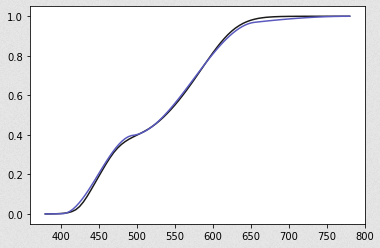Blue line is the approximated "smoothstep CDF"Black line is the original CDF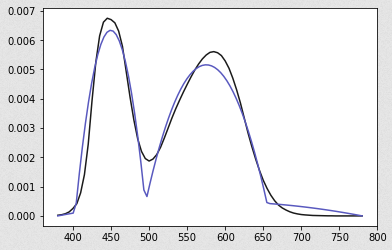Blue line is the approximated "smoothstep PDF"Black line is the original PDF
Although the "smoothstep CDF" looks smooth, its PDF is not (not C1 continuous at around 500nm). But it has 2 peaks value at around 450nm  and 600nm, may be let's try to render some images to see how it behaves.

Result

The same Sponza scene is rendered with 3 different wavelength sampling functions: uniform, cosh and smoothstep (with stratification of random number disabled, color noise are more noticeable when zoomed in):

Both cosh and smoothstep wavelength sampling method show less color noise than uniform sampling method, with the smoothstep PDF slightly better than cosh function. Seems the C1 discontinuity of the PDF does not affect rendering very much. A demo can be downloaded here to see how it looks in real-time.

Conclusion

This post described an approximated function to importance sample the visible wavelength by using the sum of the color matching functions, which reduce the color noise slightly. The approximated CDF is composed of cubic piecewise functions. The python code used for generating the polynomial coefficients can be found here (with some unused testing code too. e.g. I have tried to use 1 linear base function with 2 smaller smoothstep functions added on top, but the result is not much better...). Although the approximated PDF is not C1 continuous, it does not affect the rendering very much. If someone knows more about how the C1 continuity of the PDF affect rendering, please leave a comment below. Thank you.

References

 https://stackoverflow.com/questions/13328676/c-solving-cubic-equations